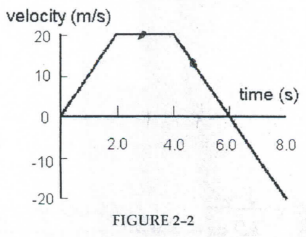Physics Practice Problems Velocity & Acceleration Graphs Practice Problems Solution: Figure 2.2 shows the velocity time graph of a moto...

🤓 Based on our data, we think this question is relevant for Professor Graf's class at SUNY.

# Solution: Figure 2.2 shows the velocity time graph of a motorist during a 8 second period. The motorist leaves home (position x = 0m) at time t = 0s and the velocity change as shown. What is the acceleration at 3.0 s?

###### Problem

Figure 2.2 shows the velocity time graph of a motorist during a 8 second period. The motorist leaves home (position x = 0m) at time t = 0s and the velocity change as shown. What is the acceleration at 3.0 s?Velocity & Acceleration Graphs

Velocity & Acceleration Graphs

#### Q. A common graphical representation of motion along a straight line is the v vs. t graph, that is, the graph of (instantaneous) velocity as a function o...

Solved • Tue Mar 24 2020 14:38:08 GMT-0400 (EDT)

Velocity & Acceleration Graphs

#### Q. (a) Determine the magnitude of the acceleration for the speeding up phase. Express your answer to two significant figures and include the appropriate ...

Solved • Wed Mar 11 2020 14:59:59 GMT-0400 (EDT)

Velocity & Acceleration Graphs

#### Q. The following three questions are related to the four velocity versus time graphs (1-4) given in the figure. The answer to each question is two of the...

Solved • Wed Mar 11 2020 12:01:31 GMT-0400 (EDT)

Velocity & Acceleration Graphs

#### Q. (a) Which graphs represent an object moving in the negative direction?(b) Which graphs represent an object that is speeding up?(c) Which graphs repres...

Solved • Wed Mar 11 2020 11:06:08 GMT-0400 (EDT)